Win up to 100% scholarship on Aakash BYJU'S JEE/NEET courses with ABNAT Win up to 100% scholarship on Aakash BYJU'S JEE/NEET courses with ABNAT

# Qualitative Analysis of Organic Compounds

## What Is Qualitative Analysis?

Quantitative analysis is an analysis method used to determine the number of elements or molecules produced during a chemical reaction.  Organic compounds comprise carbon, hydrogen, oxygen, nitrogen, phosphorus, sulphur and halogens. The various methods used for the measurement of the percentage composition of elements in an organic compound are explained here.

Qualitative analysis is the analysis of the species present in a given compound. For example, if a compound is taken, the qualitative analysis would be more focused on finding the elements and the ions present in the compound rather than study as to how much they are present.

## Detection of C and H

C and H are detected by heating the compound with CuO in a dry test tube. They are oxidised to CO2 and H2O, respectively. If the CO2 turns lime water milky, and H2O turns anhydrous CuSO4 blue, then the presence of C and H is confirmed.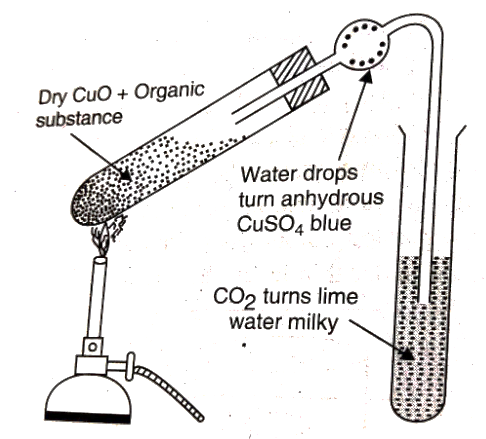Detection of Carbon and Hydrogen

## Test for Phosphorous

The organic compound is heated with an oxidising agent to oxidise phosphorous to phosphate. The solution is then boiled with concentrated HNO3 and treated with ammonium molybdate. Yellow precipitate confirms the presence of phosphorous.

The reaction is given below:

Na3PO4 + 3HNO3 → H3PO4 + 3NaNO3

H3PO4 + 12(NH4)2MoO4 + 21HNO3 → (NH4)3PO4.12MoO3 + 21NH4NO3 + 12H2O

Quantitative analysis is more towards finding out how much of the elements are present, that is, their amounts.

## Estimation of C and H

### Liebig’s Combustion Method

A known mass of the compound is heated with CuO. The carbon present is oxidised to CO2 and hydrogen to H2O. The CO2 is absorbed in the KOH solution, while H2O is absorbed by anhydrous CaCl2, and they are weighed.

Percentage of C = 12/44 x ((Mass of CO2)/(Mass of compound)) x 100

Percentage of H = 2/18 x ((Mass of H2O)/(Mass of compound)) x 100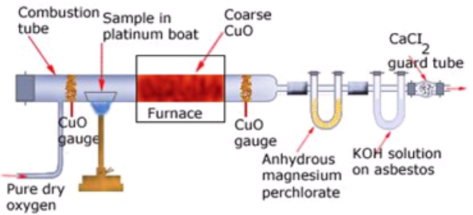Liebig’s combustion method

## Estimation of Halogens

### Carius Method

A known mass of the compound is heated with Conc. HNO3 in the presence of AgNO3 in a hard glass tube called the Carius tube. C and H are oxidised to CO2 and H2O. The halogen forms the corresponding AgX. Then, it is filtered, dried and weighed.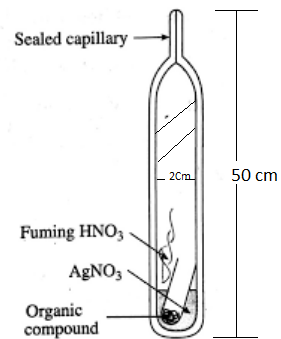Estimation of Halogens by Carius method

Percentage of X =

((Atomic mass of X)/(Molecular mass of AgX))x((Mass of AgX)/(Mass of the compound))x100

Calculations:

Let the mass of the given organic compound be m g.

Suppose the mass of AgX formed = m1 g.

We know that 1 mol of AgX consists of 1 mol of X.

So, in m1 g of AgX , mass of halogen

$$\begin{array}{l} =\frac {(atomic~mass~of~X~×~m_1~g)}{(molecular~mass~of~AgX)}\end{array}$$

Percentage of halogen

$$\begin{array}{l} =\frac {(atomic~mass~of~X~×~m_1~×~100)}{(molecular~mass~of~AgX~×~m)}\end{array}$$

## Estimation of Sulphur

A known mass of the compound is heated with conc. HNO3 in the presence of BaCl2 solution in the Carius tube. Sulphur is oxidised to H2SO4 and precipitated as BaSO4. It is then dried and weighed.

Percentage of S = ((Atomic mass of S)/(Molecular mass of BaSO4)) x (( Mass of BaSO4)/(Mass of the compound)) x 100

Calculations:

Suppose the mass of organic compound = mg

Let the mass of barium sulphate formed = m1 g

We know that 32 g sulphur is present in 1 mol of BaSO4

Therefore, 233 g BaSO4 contains 32 g sulphur:

$$\begin{array}{l}\Rightarrow M_1\ g\ \text{of}\ BaSO_4\ \text{contains}\ \frac {32~×~m_1}{233} g\ \text{of sulphur}\end{array}$$

Percentage of sulphur

$$\begin{array}{l} =\frac {32~×~m_1~×~100}{233~×~m}\end{array}$$

## Estimation of Phosphorus

A known mass of the compound is heated with HNO3 in a Carius tube, which oxidises phosphorous to phosphoric acid. It is then precipitated as ammonium phosphomolybdate ((NH4)3PO4.12MoO3) by adding NH3 and ammonium molybdate ((NH4)2MoO4). Then, it is filtered, dried and weighed.

Percentage of P=

((Atomic mass of P)/(Molecular mass of (NH4)3PO4.12MoO3)) x ((Mass of (NH4)3PO4.12MoO3)/(Mass of compound)) x 100

## Estimation of Nitrogen

### Estimation of Nitrogen by Dumas Method

A known mass of the compound is heated with CuO in an atmosphere of CO2, which yields free nitrogen along with CO2 and H2O.

CxHyNz + (2x+ 0.5y) CuO → xCO2 + 0.5y H2O + 0.5z (N2) + (2x+ 0.5y)Cu

The gases are passed over a hot copper gauze to convert trace amounts of nitrogen oxides to N2. The gaseous mixture is collected over a solution of KOH, which absorbs CO2, and nitrogen is collected in the upper part of the graduated tube.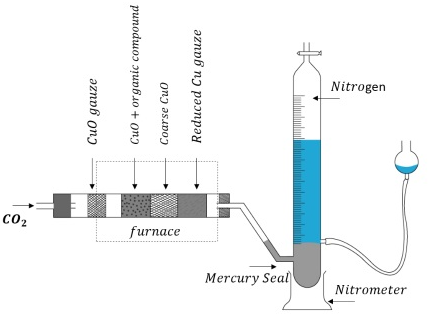Estimation of Nitrogen by Dumas Method

Let the volume of N2 collected be V1 mL.

Then, volume of N2 at STP = (P1V1 x 273)/ (760 x T1) = V mL

Where P1 and V1 are the pressure and volume of N2.

P1= Atmospheric pressure – aqueous tension

22.4 L of N2 weighs 28 g,

Therefore, V ml of N2 weighs

=(28 x V)/22400 grams

The percentage of N would be,

= (28/22400) x (V/ Mass of compound) x 100.

### Estimation of Nitrogen by Kjeldahl Method

A known mass of an organic compound (0.5 g) is mixed with K2SO4 (10 g), CuSO4 (1.0 g) and conc.H2SO4 (25 mL), and heated in a Kjeldahl’s flask.

CuSO4 acts as a catalyst, while K2SO4 raises the boiling point of sulphuric acid. The nitrogen in the compound is quantitatively converted to (NH4)2SO4. The resulting mixture is reacted with excess of NaOH solution, and the NH3, so evolved, is passed into a known but excess volume of standard acid.

The acid left unreacted is estimated by titration with some standard alkali. Thus, the percentage of nitrogen can be calculated.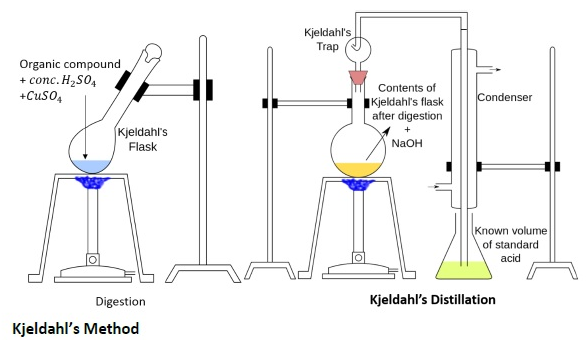Estimation of Nitrogen by Kjeldahl Method

The reactions are given below:

1. C + H + S → CO2 + H2O + SO2
2. N → (NH4)2SO4
3. (NH4)2SO4 + 2NaOH → Na2SO4 + 2NH3 + 2H2O
4. 2NH3 + H2SO4 → (NH4)2SO4

Calculation of the percentage of N

Let the mass of the organic compound be mg.

Volume of H2SO4 (Molarity M) = V mL

The volume of NaOH of molarity M used for titration excess of H2SO4 = V1 mL

mEq of excess H2SO4 = mEq of NaOH

= MV1 mEq

Total mEq of H2SO4 taken = 2MV

mEq of H2SO4 used for neutralisation of NH3 = (2MV – MV1)

Therefore,

mEq of NH3 = (2MV-MV1)

1000 mEq or 1000 mL of NH3 solution contains = 17 g of NH3 (or) 14 g of N

Therefore,

(2MV-MV1) mEq of NH3 contains

=(14 x (2MV-MV1)) / 1000 g of N

Percentage of N= ((14x(2MV-MV1)) x (100/(1000xm)))

## Qualitative Analysis of Organic Compounds – Kjeldahl’s Method## Estimation of Oxygen by Aluise’s Method

A known mass of the compound is decomposed by heating it in the presence of N2 gas. The mixture of gases so produced is passed over red hot coke. This is done so that all the O2 is converted to CO. This mixture is heated with I2O5, in which CO is oxidised to CO2, liberating I2.

The reactions are given below:

Organic compound → Other gaseous products + O2

2C + O2 → 2CO

I2O5 + 5CO → 5CO2 + I2

Percentage of O = ((Molecular mass of O2/Molecular mass of CO2) x (Mass of CO2/Mass of the compound) x 100

Q1

### What is meant by qualitative analysis?

Qualitative analysis determines the percentage composition of elements present in a compound.
Q2

### Which method is used to estimate carbon and hydrogen?

The Liebig’s combustion method is used to estimate carbon and hydrogen. The hydrogen present is oxidised to water, and the carbon present is oxidised to carbon dioxide.
Q3

### Which method is used for the estimation of halogens? The estimation of halogen is done by the Carius method. Halogens form a precipitate with silver nitrate.

The lower reduction potential of an element implies that the element gets oxidised easily.
Q4

### Which method is used for the estimation of nitrogen?

The Dumas method is used for the estimation of hydrogen. Nitrogen gas is released in its free form, confirming the presence of nitrogen.
Q5

### What is the catalyst used in Kjeldahl’s method for the estimation of nitrogen?

CuSO4 is used as a catalyst for the estimation of nitrogen in Kjeldahl’s method.

Test your Knowledge on Organic compounds